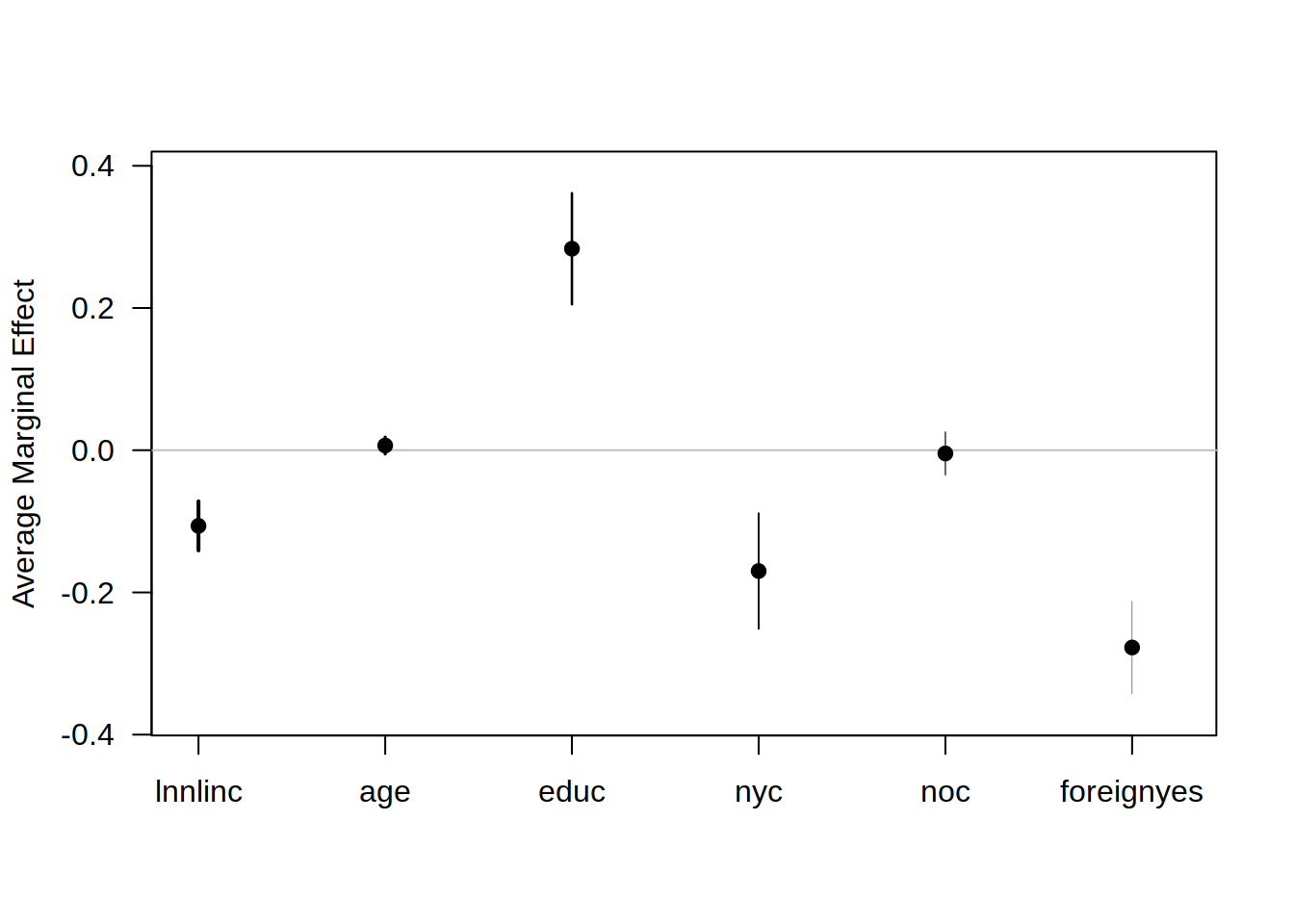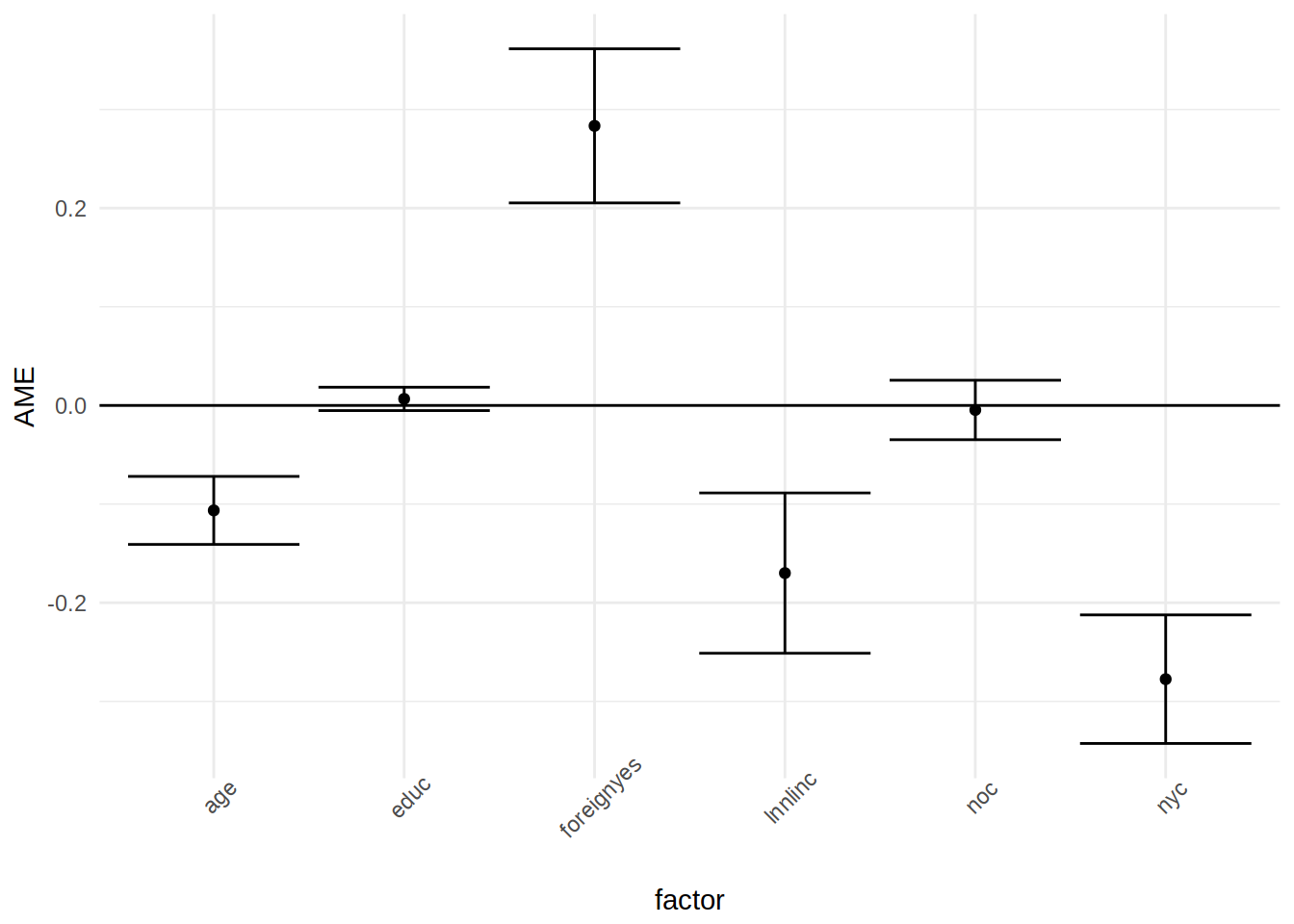Have a question about one of the blog posts? Chat here with me.

# Easy peasy STATA-like marginal effects with R

R

Model interpretation is essential in the social sciences. If one wants to know the effect of variable `x` on the dependent variable `y`, marginal effects are an easy way to get the answer. STATA includes a `margins` command that has been ported to R by Thomas J. Leeper of the London School of Economics and Political Science. You can find the source code of the package on github. In this short blog post, I demo some of the functionality of `margins`.

``````library(ggplot2)
library(tibble)
library(broom)
library(margins)
library(Ecdat)``````

As an example, we are going to use the `Participation` data from the `Ecdat` package:

``data(Participation)``
``?Participation``
``````Labor Force Participation

Description

a cross-section

number of observations : 872

observation : individuals

country : Switzerland

Usage

data(Participation)
Format

A dataframe containing :

lfp
labour force participation ?

lnnlinc
the log of nonlabour income

age
age in years divided by 10

educ
years of formal education

nyc
the number of young children (younger than 7)

noc
number of older children

foreign
foreigner ?

Source

Gerfin, Michael (1996) “Parametric and semiparametric estimation of the binary response”, Journal of Applied Econometrics, 11(3), 321-340.

References

Davidson, R. and James G. MacKinnon (2004) Econometric Theory and Methods, New York, Oxford University Press, http://www.econ.queensu.ca/ETM/, chapter 11.

Journal of Applied Econometrics data archive : http://qed.econ.queensu.ca/jae/.``````

The variable of interest is `lfp`: whether the individual participates in the labour force or not. To know which variables are relevant in the decision to participate in the labour force, one could estimate a logit model, using `glm()`.

``logit_participation = glm(lfp ~ ., data = Participation, family = "binomial")``

Now that we ran the regression, we can take a look at the results. I like to use `broom::tidy()` to look at the results of regressions, as `tidy()` returns a nice `data.frame`, but you could use `summary()` if you’re only interested in reading the output:

``tidy(logit_participation)``
``````##          term    estimate  std.error  statistic      p.value
## 1 (Intercept) 10.37434616 2.16685216  4.7877499 1.686617e-06
## 2     lnnlinc -0.81504064 0.20550116 -3.9661122 7.305449e-05
## 3         age -0.51032975 0.09051783 -5.6378920 1.721444e-08
## 4        educ  0.03172803 0.02903580  1.0927211 2.745163e-01
## 5         nyc -1.33072362 0.18017027 -7.3859224 1.514000e-13
## 6         noc -0.02198573 0.07376636 -0.2980454 7.656685e-01
## 7  foreignyes  1.31040497 0.19975784  6.5599678 5.381941e-11``````

From the results above, one can only interpret the sign of the coefficients. To know how much a variable influences the labour force participation, one has to use `margins()`:

``````effects_logit_participation = margins(logit_participation)

print(effects_logit_participation)``````
``## Average marginal effects``
``## glm(formula = lfp ~ ., family = "binomial", data = Participation)``
``````##  lnnlinc     age     educ     nyc       noc foreignyes
##  -0.1699 -0.1064 0.006616 -0.2775 -0.004584     0.2834``````

Using `summary()` on the object returned by `margins()` provides more details:

``summary(effects_logit_participation)``
``````##      factor     AME     SE       z      p   lower   upper
##         age -0.1064 0.0176 -6.0494 0.0000 -0.1409 -0.0719
##        educ  0.0066 0.0060  1.0955 0.2733 -0.0052  0.0185
##  foreignyes  0.2834 0.0399  7.1102 0.0000  0.2053  0.3615
##     lnnlinc -0.1699 0.0415 -4.0994 0.0000 -0.2512 -0.0887
##         noc -0.0046 0.0154 -0.2981 0.7656 -0.0347  0.0256
##         nyc -0.2775 0.0333 -8.3433 0.0000 -0.3426 -0.2123``````

And it is also possible to plot the effects with base graphics:

``plot(effects_logit_participation)``This uses the basic R plotting capabilities, which is useful because it is a simple call to the function `plot()` but if you’ve been using `ggplot2` and want this graph to have the same look as the others made with `ggplot2` you first need to save the summary in a variable. Let’s overwrite this `effects_logit_participation` variable with its summary:

``effects_logit_participation = summary(effects_logit_participation)``

And now it is possible to use `ggplot2` to create the same plot:

``````ggplot(data = effects_logit_participation) +
geom_point(aes(factor, AME)) +
geom_errorbar(aes(x = factor, ymin = lower, ymax = upper)) +
geom_hline(yintercept = 0) +
theme_minimal() +
theme(axis.text.x = element_text(angle = 45))``````So an infinitesimal increase, in say, non-labour income (`lnnlinc`) of 0.001 is associated with a decrease of the probability of labour force participation by 0.001*17 percentage points.

You can also extract the marginal effects of a single variable, with `dydx()`:

``head(dydx(Participation, logit_participation, "lnnlinc"))``
``````##   dydx_lnnlinc
## 1  -0.15667764
## 2  -0.20014487
## 3  -0.18495109
## 4  -0.05377262
## 5  -0.18710476
## 6  -0.19586986``````

Which makes it possible to extract the effects for a list of individuals that you can create yourself:

``````my_subjects = tribble(
~lfp,  ~lnnlinc, ~age, ~educ, ~nyc, ~noc, ~foreign,
"yes",   10.780,  7.0,     4,    1,    1,    "yes",
"no",     1.30,  9.0,     1,    4,    1,    "yes"
)

dydx(my_subjects, logit_participation, "lnnlinc")``````
``````##   dydx_lnnlinc
## 1  -0.09228119
## 2  -0.17953451``````

I used the `tribble()` function from the `tibble` package to create this test data set, row by row. Then, using `dydx()`, I get the marginal effect of variable `lnnlinc` for these two individuals. No doubt that this package will be a huge help convincing more social scientists to try out R and make a potential transition from STATA easier.